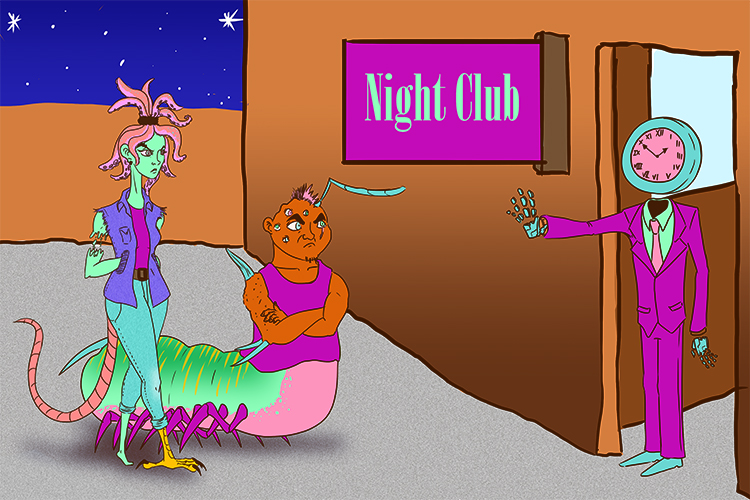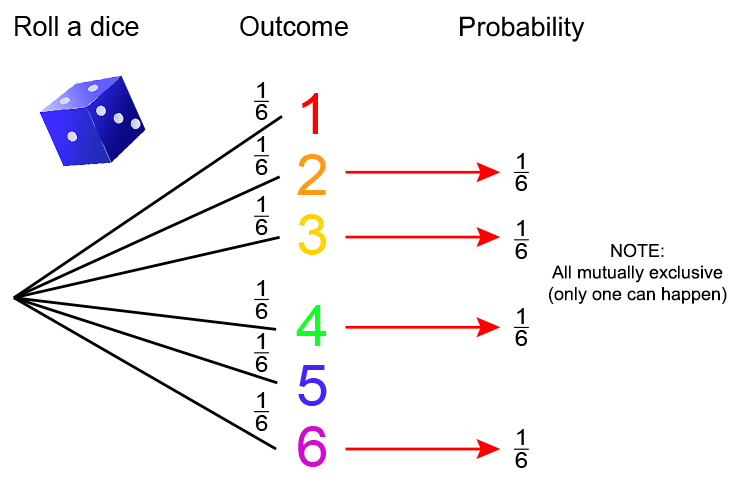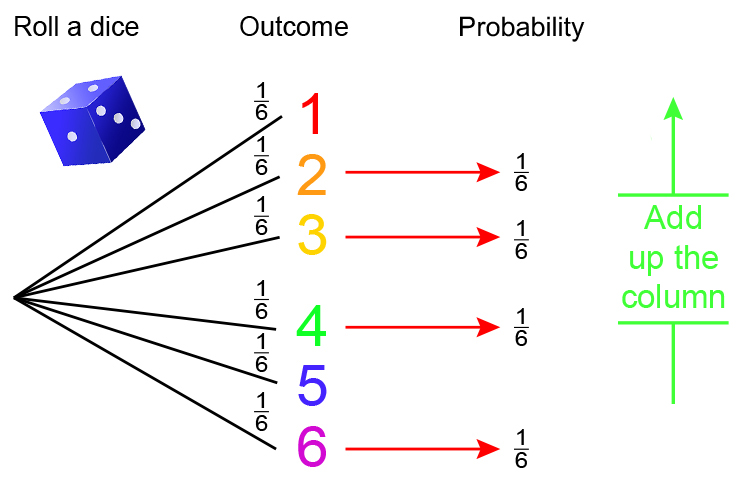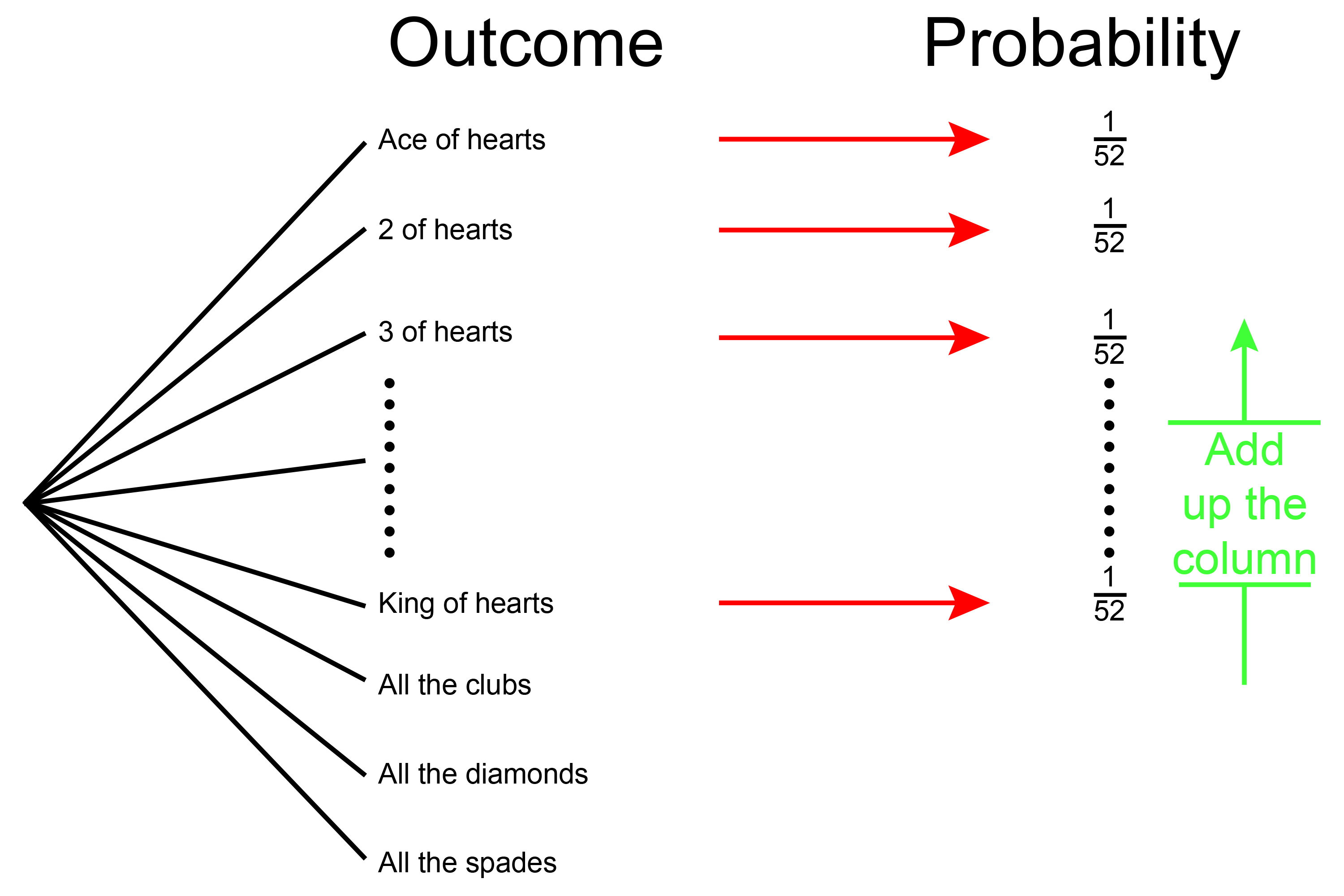# Mutually exclusive

Time – can’t both happen (at the same time)Two mutants (mutually) are excluded (excluded) from entering the club together by time.

Mutually exclusive is about time. The event can’t happen at the same time.

E.g. you can’t have both 1 and 2 children. Throwing a dice and obtaining a 6 and a 1 are mutually exclusive. You can’t be 170cm and 180cm tall.

Example 1

If you roll a dice, what is the probability of rolling a 3 or rolling an even number? (This is a mutually exclusive example.)

Remember\ probability\=(N\umber\ of\ RIGHT\ answers)/(N\umber\ of \ALL\ possibl\e\ answers

Start by drawing a probability tree:Now add the probabilities up the column.1/6+1/6+1/6+1/6=(1+1+1+1)/6=4/6=2/3

The probability of rolling a dice and getting a three or an even number is 2 in 3 or = 0.67.

And if 1 = 100%  then 0.67 = 67% chance.

Example 2

What is the probability of you picking a heart from a pack of cards?

Remember\ probability\=(N\umber\ of\ RIGHT\ answers)/(N\umber\ of \ALL\ possibl\e\ answers

For 1 card probability = 1/52

Start by drawing a probability tree:1/52+1/52+1/52+1/52+1/52+1/52+1/52+1/52+1/52+1/52+1/52+1/52+1/52=13/52

The probability of picking a heart from a pack of cards = 13/52=1/4